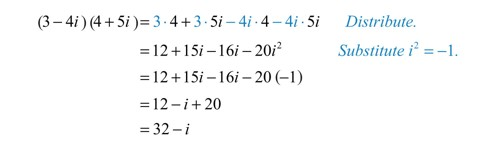Mathematic

# Multiplication of Two Complex NumbersA complex number is a number of the form a + bi, where ‘a’ and ‘b’ are real numbers and ‘I’ is an indeterminate satisfying i2 = −1. For example, 2 + 3i is a complex number.

The equations x2 + 5 = 0, x2 + 10 = 0, x2 = -1 are not solvable in the real number system i.e, these equations has no real roots. For example, i is the solution of the equation x2 = -1 and it has two solutions i.e., x = ± i, where √-1.

The number, ‘I’ is called an imaginary number. Generally, the square root of any negative real number is called imaginary number. The concept of imaginary numbers was first introduced by mathematician “Euler”. He was the one who introduced ‘i’ (read as ‘iota’) to represent √-1. He also defined i2 = -1.

Multiplication of Two Complex Numbers

Multiplication of two complex numbers is also a complex number.

In other words, the product of two complex numbers can be expressed in the standard form A + iB where A and B are real.

Let z1 = p + iq and z2 = r + is be two complex numbers (p, q, r and s are real), then their product z1z2 is defined as

z1z2 = (pr – qs) + i(ps + qr).

Proof:

Given z1 = p + iq and z2 = r + is

Now, z1z2 = (p + iq)(r + is) = p(r + is) + iq(r + is) = pr + ips + iqr + i2qs

We know that i2 = -1. Now putting i2 = -1 we get,

= pr + ips + iqr – qs

= pr – qs + ips + iqr

= (pr – qs) + i(ps + qr).

Thus, z1z2 = (pr – qs) + i(ps + qr) = A + iB where A = pr – qs and B = ps + qr are real.

Therefore, product of two complex numbers is a complex number.

Note: Product of more than two complex numbers is also a complex number.

For example:

Let z1 = (4 + 3i) and z2 = (-7 + 6i), then

z1z2 = (4 + 3i)(-7 + 6i)

= 4(-7 + 6i) + 3i(-7 + 6i)

= -28 + 24i – 21i + 18i2

= -28 + 3i – 18

= -28 – 18 + 3i

= -46 + 3iExample: Multiplication of Two Complex Numbers

Properties of multiplication of complex numbers:

If z1, z2 and z3 are any three complex numbers, then

(i) z1z2 = z2z1 (commutative law)

(ii) (z1z2)z3 = z1(z2z3) (associative law)

(iii) z ∙ 1 = z = 1 ∙ z, so 1 acts as the multiplicative identity for the set of complex numbers.

(iv) Existence of multiplicative inverse

For every non-zero complex number z = p + iq, we have the complex number p / (p2+q2) – i q / (p2+q2) (denoted by z-1 or 1/z) such that

z ∙ 1/z = 1 = 1/z ∙ z (check it)

1/z is called the multiplicative inverse of z.

Note: If z = p + iq then z-1 = 1 / (p+iq) = 1/(p+iq) ∙ (p−iq)(p−iq) = (p−iq)/(p2+q2)

(v) Multiplication of complex number is distributive over addition of complex numbers.

If z1, z2 and z3 are any three complex numbers, then

z1(z2 + z3) = z1 z2 + z1z3

and (z1 + z2)z3 = z1z3+ z2z3

The results are known as distributive laws.

Example

Find the product of two complex numbers (-2 + √3i) and (-3 + 2√3i) and express the result in standard from A + iB.

Solution:

(-2 + √3i)(-3 + 2√3i)

= -2(-3 + 2√3i) + √3i(-3 + 2√3i)

= 6 – 4√3i – 3√3i + 2(√3i)2

= 6 – 7√3i – 6

= 6 – 6 – 7√3i

= 0 – 7√3i, which is the required form A + iB, where A = 0 and B = – 7√3

http://www.math-only-math.com/multiplication-of-two-complex-numbers/